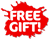Skip to content

# Texas Instruments MultiView Yellow Teacher Kit - 30XSMV/TKT/1L1/B

Condition: New
Sold out
\$199.99
\$199.99 - \$199.99
Current price \$199.99
SKU TEX30XSMVTKT1L1B##### Item Description
TI-30XS MultiView EZ Spot Calculator. TTeacher Kit with EZ Spot yellow calculators with School Property on the front. Teacher Kit includes ten (10) calculators, one (1) storage caddy, one (1) poster, and one (1) set of instructional materials.

## Overview

View more calculations at a time

Four-line display allows you to enter more than one calculation; compare results and explore patterns; all on the same screen.

MathPrint feature

Use the MathPrint feature to display expressions; symbols; and fractions just as they appear in textbooks.

Scientific notation output

View scientific notation with the proper superscripted exponents and see the output in scientific notation.

Explore (x;y) table of values

Students can easily explore an (x;y) table of values for a given function; automatically or by entering specific x values.

Product Features:

• Four-line display
• One- and two-variable statistics
• MathPrint feature
• Fraction/decimal conversion
• Step-by-step fraction simplification
• Edit; cut and paste entries
• Solar and battery powered

Specifications:

Built-in functionality:

• Review and edit previous entries via a scrollable home screen
• Paste inputs or outputs into new calculations
• MathPrint entry and output mode for viewing calculations in math notation; including answers in terms of π; square roots and fraction
• CLASSIC mode for similar entry and compatibility with previous two-line scientifics
• Symbolic notation of π
• Toggle key to change the form of answers between exact and decimal approximation
• Stacked fractions and fraction functions
• Fraction/decimal/percent conversions
• Change between improper fractions and mixed numbers
• Automatic simplification of fractions
• Random number and random integer generator
• Central MODE menu for selecting calculator mode settings

View more calculations at a time

Four-line display allows you to enter more than one calculation; compare results and explore patterns; all on the same screen.

MathPrint feature

Use the MathPrint feature to display expressions; symbols; and fractions just as they appear in textbooks.

Scientific notation output

View scientific notation with the proper superscripted exponents and see the output in scientific notation.

Explore (x;y) table of values

Students can easily explore an (x;y) table of values for a given function; automatically or by entering specific x values.

Product Features:

• Four-line display
• One- and two-variable statistics
• MathPrint feature
• Fraction/decimal conversion
• Step-by-step fraction simplification
• Edit; cut and paste entries
• Solar and battery powered

Specifications:

Built-in functionality:

• Review and edit previous entries via a scrollable home screen
• Paste inputs or outputs into new calculations
• MathPrint entry and output mode for viewing calculations in math notation; including answers in terms of π; square roots and fraction
• CLASSIC mode for similar entry and compatibility with previous two-line scientifics
• Symbolic notation of π
• Toggle key to change the form of answers between exact and decimal approximation
• Stacked fractions and fraction functions
• Fraction/decimal/percent conversions
• Change between improper fractions and mixed numbers
• Automatic simplification of fractions
• Random number and random integer generator
• Central MODE menu for selecting calculator mode settings
• Functions accessed directly through keys or through pull-down menus
• Negation key
• One constant operator feature
• Combinations and permutations
• Trigonometry
• Hyperbolics
• Logs and antilogs
• %; x; /x; yˆx; π; x!
• Fixed decimal capability
• (x;y) table feature with Auto and Ask-x options
• Basic Data/List Editor with three lists
• List formulas
• One- and two-variable statistics with permanent stat variable input storage
• EOS: Equation Operating System
• Up to eight pending operations
• Up to 23 levels of parentheses
• Error recovery capability
• Auto Power Off
• Quick/easy reset of calculator via 2 key press or menu for exam purposes
• Seven memory variables. (x;y;z;t;a;b;c)
• Scientific and engineering notation
• x10n key for quick input of numbers in powers of 10

Hardware:

• Four-line × 16-character; easy-to-read LCD display
• Dual power (solar and battery)
• Hard plastic; color-coded keys
• Non-skid rubber feet
• Snap-on protective hard case
• Teacher kit includes 10 calculators; storage caddy; Teacher's Guide in English and Spanish; calculator poster and transparency
• Quick-reference card

Show Less
x